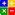# Ratio - Average Days Delinquent (ADD)AVERAGE DAYS DELINQUENT (ADD):Online ADD Ratio Calculator

Average Days Delinquent (ADD) is a measure of the average number of days that it takes for an Invoice to get paid.

It is one of the collection metrics that measures collection effort and effectiveness.

Formula:

ADD = Regular DSO – Best Possible DSO

Where DSO stands for 'Days Sales Outstanding'

Since the formulas for ADD & DSO have common elements, if one increases the other one should also increase and vice versa.

Your collection efforts will impact both measures favourably or unfavourably

Example:

First the Formulas:

Average Days Delinquent = Regular DSO – Best Possible DSO

Where:

Regular DSO (DSO) = (Total Accounts Receivables/Total Credit Sales) x Number of Days in the period that is being analyzed

Best Possible DSO = (Current Receivables/Total Credit Sales)  x Number of Days in the period that is being analyzed

Data:

Total Accounts Receivable = \$15,000
Current Accouts Receivable = \$4,000
Credit Sales made in a 30 days period = \$10,000

First Calculate the Regular DSO:
Regular DSO or (DSO) = (Total Accounts Receivables/Total Credit Sales) x Number of Days in the period that is being analyzed
Regular DSO = (15,000/10,000) x 30
Regular DSO = 45 Days

Then Calculate 'Best Possible DSO':
Best Possible DSO = (Current Receivables/Total Credit Sales) x Number of Days in the period that is being analyzed
Best Possible DSO = (4,000/10,000) x 30
Best Possible DSO = 12 Days

Now Calculate Average Days Delinquent (ADD)
Average Days Delinquent = Regular DSO – Best Possible DSO